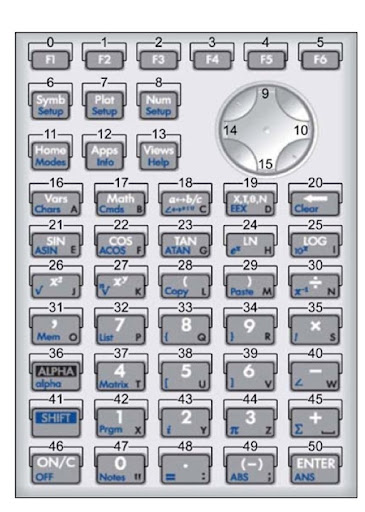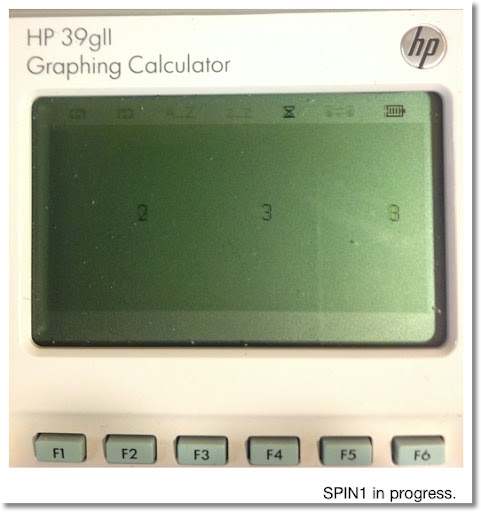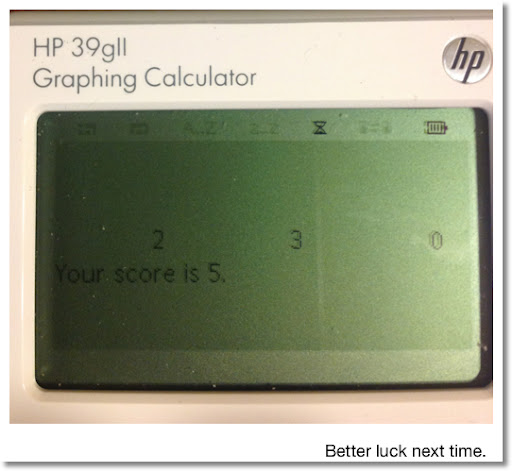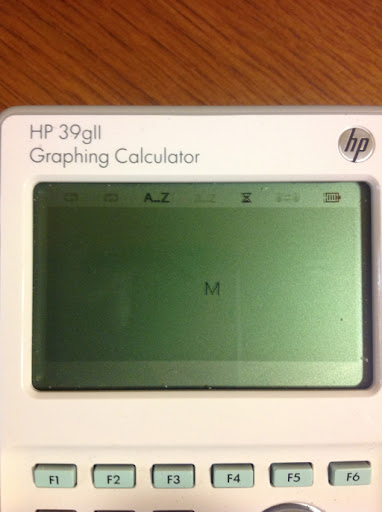## Monday, April 1, 2013

### HP 39gii Programming Tutorial Part 6: Making programs interactive with GETKEY

Welcome to Part 6 of the HP 39gii programming tutorial. In today's session, it is all about GETKEY. The GETKEY command allows programs to be interactive during execution.

GETKEY and Key Codes

Keystroke sequence for GETKEY:
[SHIFT] [Math] [ F1 ] [ 5 ] [ 5 ]

GETKEY returns the key number of the last key pressed. If there has not been a key pressed, GETKEY returns -1.

GETKEY assigns a key code to each of the keys, starting with 1 on the upper left corner of the keyboard, the [ F1 ], going right then down, to the lower right corner of the keyboard, [ENTER], which has code 50.

The key codes for the arrow keys are as follows:
[ up ] : 9
[ right ] : 10
[ left ] : 14
[ right ] : 15

Below is the map of key codes. (Source: HP 39gii User's Guide, Edition 1)How to use GETKEY

In today's tutorial, we will demonstrate two uses of GETKEY:

1. Execute commands until any key is pressed.

General structure:
WHILE GETKEY==-1 DO
(Commands)
END;

2. Assign certain actions to certain keys.

Let E be the code for the exit key. Press the key that has the corresponding code E, and the loop ends.

REPEAT
...
GETKEY:=K;
....
IF K==(number) (and/or other conditions) THEN
(do this); END;
...
UNTIL K==E;

Let's demonstrate this in a couple of programs.

The Program SPIN1

Spinning Game #1: Use GETKEY to repeat an action until a key (any key is pressed)

Three spinners spin between 0 and 4. Press any key at any time and try to get the highest score possible.

EXPORT SPIN1()
BEGIN
RANDSEED();
RECT();
WAIT(0.5);
WHILE GETKEY==-1 DO
RECT();
INT(RANDOM(0,5))→A;
INT(RANDOM(0,5))→B;
INT(RANDOM(0,5))→C;
TEXTOUT_P(string(A),60,53);
TEXTOUT_P(string(B),145,53);
TEXTOUT_P(string(C),230,53);
WAIT(0.25);
END;
A+B+C→S;
WAIT(0);
END;The MOVEM Program

The program MOVEM uses GETKEY to make an interactive program. Use the arrow keys to move the "M" character around. Exit the program with by pressing the [ F6 ] key.

The characters of the large font has a size of 10 x 12 pixels.

To erase a character at a position, use
TEXTOUT_P(character, x, y, font size, 3)

The last 3 tells the 39gii to type the character in white. This is necessary to give the "M" movement in MOVEM.

EXPORT MOVEM()
BEGIN
RECT();
A:=100;
B:=60;
REPEAT
TEXTOUT_P("M",A,B,2);
K:=GETKEY;
IF K≠1 THEN
TEXTOUT_P("M",A,B,2,3);
END;
IF K==14 AND A>0 THEN
A:=A-10; END;
IF K==10 AND A<240 THEN
A:=A+10; END;
IF K==15 AND B<108 THEN
B:=B+12; END;
IF K==9 AND B>0 THEN
B:=B-12; END;
UNTIL K==5;
END;

The screen shot below shows MOVEM in action. The can be a base for a simple game.Next time, in Part 7, we'll show you how to use App commands in a program.

This blog is property of Edward Shore. 2013

### RCL 40 Book On Sale

RCL 40 Book On Sale The fantastic book, RCL 40: Recollection, Reinvention, & HP Calculators , edited by W.A.C. Mier-Jedrezejowic, Ph. D,...